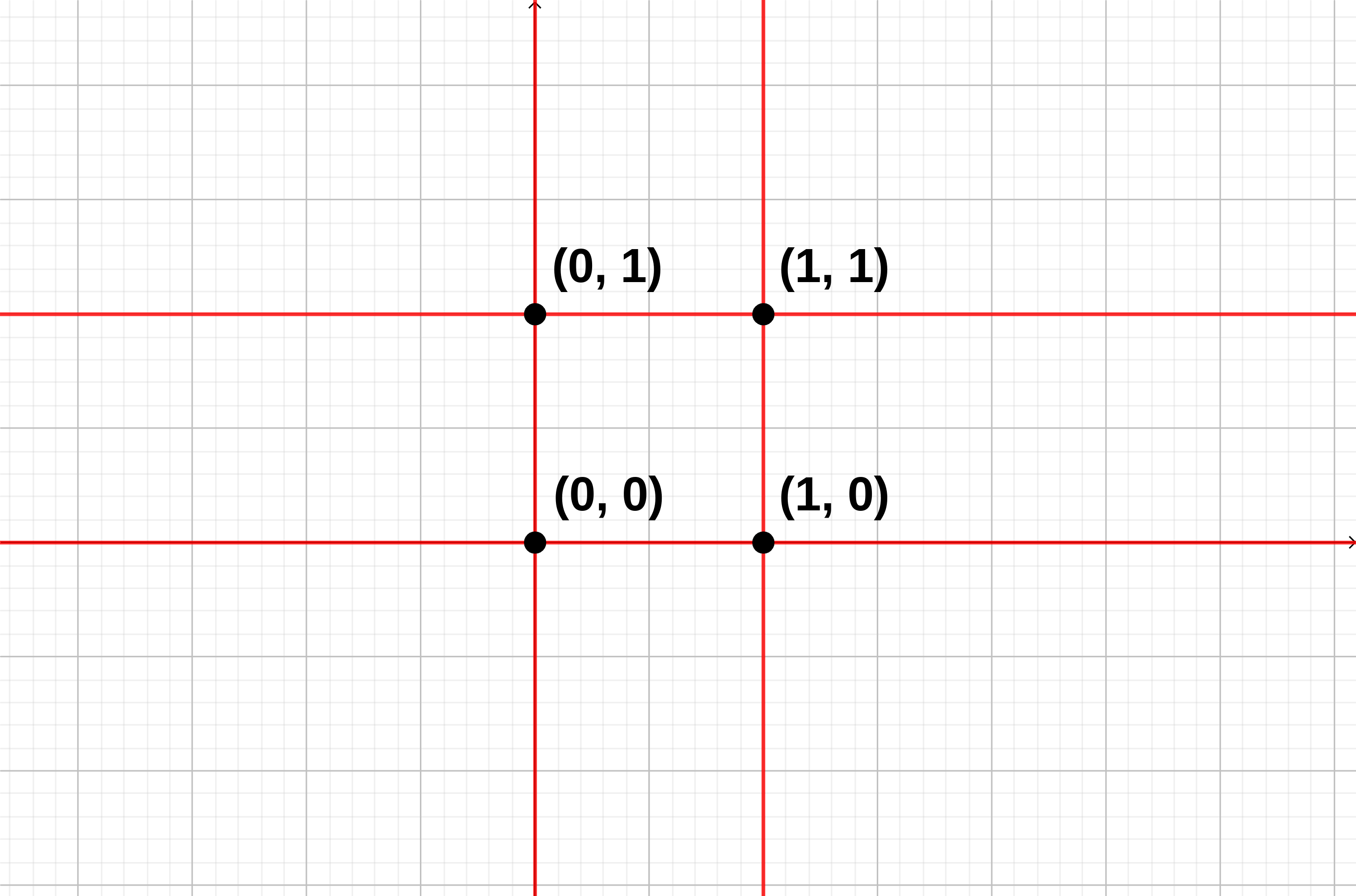# Points and Lines codechef

Points and Lines codechef Question:-

Given N points of the form (xi,yi) on a 2-D plane.

From each point, you draw 22 lines one horizontal and one vertical. Now some of the lines may overlap each other, therefore you are required to print the number of distinct lines you can see on the plane.

Note:

• Two horizontal lines are distinct if they pass through different yy coordinates.
• Two vertical lines are distinct if they pass through different xx coordinates.

### Input Format

• First line will contain TT, number of testcases. Then the testcases follow.
• Each testcase contains a single integer NN, the number of points.
• The next NN lines contain two space separated integers xi,yixi,yi, the coordinate of the ithith point.

### Output Format

For each testcase, output in a single line the number of distinct lines that can be seen on the plane.

### Constraints

• 1T10001≤T≤1000
• 1N1051≤N≤105
• 0Xi,Yi1090≤Xi,Yi≤109
• Sum of NN over all test cases is atmost 105105.

### Sample Input 1

3
4
1 1
1 0
0 1
0 0
5
0 0
0 1
0 2
0 3
0 4
1
10 10


### Sample Output 1

4
6
2


### Explanation

Test Case 11: There are 22 horizontal lines passing through Y=0Y=0 and Y=1Y=1, and 22 vertical lines passing through X=0X=0 and X=1X=1.Test Case 22: There are 55 horizontal lines passing through Y=0,Y=1,Y=2,Y=3Y=0,Y=1,Y=2,Y=3 and Y=4Y=4 and 11 vertical line passing through X=0X=0.

Test Case 33: There is 11 horizontal line passing through Y=10Y=10 and 11 vertical line passing through X=10X=10.

### Code of Points and Lines codechef:-

t=int(input())
for j in range(t):
h=[]
v=[]
ans=0
n=int(input())
for i in range(n):
e=input().split()
h.append(e)
v.append(e)
if e==1 and e==0:
ans+=1
h=list(set(h))
v=list(set(v))
ans=ans+len(h)+len(v)
print(ans)

### Points and Lines codechef

Recommended post:-